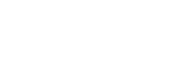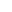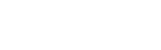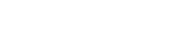Calculation Transformation Function allows end-user to write scripts to transform data. Input values represented as [F00N] Where N is a value number. For example calculation you can see above has got two input values [F001] and [F002]. The programming language used is very close to Pascal.

The Structure of a Calculation

Below is the basic structure that every Calculation transformation must follow:

Var
VariableName : VariableType;
VariableName : VariableType;
...
// Some single line Comment if necessary

{
Multi line
Comment
}

Procedure ProcedureName;

variables here if necessary
Begin

Some Code;
End;

Function FunctionName(variableList): VariableType;
variables here if necessary
Begin

Some Code if necessary;
Result := some expression
More Code if necessary;
End;

... some more functions and procedures if necessary ...

Begin

the main program block.
Result:= some calculation
End.

Note:
The functions and procedures can appear in any order. The only requirement is that if one procedure or function uses another one, that latter one must have been defined already.

Declaring Variables

var // This starts a section of variables
LineTotal: Integer; // This defines an Integer variable called LineTotal
First,Second: String; // This defines two variables to hold strings of text

Assigning Values to Variables

Variables are simply a name for a block of memory cells in main memory. If a value is assigned to a variable, that value must be of the same type as the variable, and will be stored in the memory address designated by the variable name. The assignment statement is the semicolon-equal :=.

• Variables must be declared at the beginning of the program, a procedure, or a function
• Variables must be initialized before they can be used.
• Variables can be reused as often as necessary. Their old value is simply overwritten by a new assignment.

Example:

Var
i : Integer: { variable name is i, type is integer)
Begin
i := 10; { valid integer number assigned to variable i }
End.

Variable Types

Numeric Data Types

TypeStorageSize/Range
Byte 1 0 to 255
ShortInt 1 -127 to 127
Word 2 0 to 65,535
SmallInt 2 -32,768 to 32,767
LongWord 4 0 to 4,294,967,295
Cardinal 4* 0 to 4,294,967,295
LongInt 4 -2,147,483,648 to 2,147,483,647
Integer 4* -2,147,483,648 to 2,147,483,647
Int64 8 -9,223,372,036,854,775,808 to 9,223,372,036,854,775,807
Single 4 7 significant digits, exponent -38 to +38
Currency 8 50+ significant digits, fixed 4 decimal places
Double 8 15 significant digits, exponent -308 to +308
Extended 10 19 significant digits, exponent -4932 to +4932

Assigning to and from number variables

Number variables can be assigned from other numeric variables, and expressions:

var
Age : Byte; // Smallest positive integer type
Books : SmallInt; // Bigger signed integer
Salary : Currency; // Decimal used to hold financial amounts
Expenses : Currency;
TakeHome : Currency;
begin

Expenses := 12345.67; // Assign from a literal constant
TakeHome := Salary; // Assign from another variable
TakeHome := TakeHome - Expenses; // Assign from an expression
end;

Numerical operators

Number calculations, or expressions, have a number of primitive operators available:

+ Add one number to another
- Subtract one number from another
* Multiply two numbers
/ Divide one decimal number by another
div Divide one integer number by another
mod Remainder from dividing one integer by another

When using these multiple operators in one expression, you should use round brackets to wrap around sub-expressions to ensure that the result is obtained. This is illustrated in the examples below:

var
myInt : Integer; // Define integer and decimal variables
myDec : Single;
begin
myInt := 20; // myInt is now 20
myInt := myInt + 10; // myInt is now 30
myInt := myInt - 5; // myInt is now 25
myInt := myInt * 4; // myInt is now 100
myInt := 14 div 3; // myInt is now 4 (14 / 3 = 4 remainder 2)
myInt := 14 mod 3; // myInt is now 2 (14 / 3 = 4 remainder 2)
myInt := 12 * 3 - 4; // myInt is now 32 (* comes before -)
myInt := 12 * (3 - 4); // myInt is now -12 (brackets come before *)
myDec := 2.222 / 2.0; // myDec is now 1.111
end;

Character Types

var

Str1 : Char; // Holds a single character, small alphabet
Str2 : WideChar; // Holds a single character, International alphabet
Str3 : AnsiChar; // Holds a single character, small alphabet
Str4 : ShortString; // Holds a string of up to 255 Char's
Str5 : String; // Holds strings of Char's of any size desired
Str6 : AnsiString; // Holds strings of AnsiChar's any size desired
Str7 : WideString; // Holds strings of WideChar's of any size desired

Some simple text variable usage examples are given below:

+ Concatenates two strings together
= Compares for string equality
< Is one string lower in sequence than another
<= Is one string lower or equal in sequence with another
> Is one string greater in sequence than another
>= Is one string greater or equal in sequence with another
<> Compares for string inequality

Variants

The Variant data type provides a flexible general-purpose data type.

It can hold anything but structured data and pointers.

Variants are useful in very specific circumstances, where data types and their content are determined at run time rather than at compile time.

Example:

var
myVar : Variant;

Date Variables

TDateTime

Description

The TDateTime type holds a date and time value.

It is stored as a Double variable, with the date as an integral part, and time as fractional part. The date is stored as the number of days since 30 Dec 1899. Quite why it is not 31 Dec is not clear. 01 Jan 1900 has a days value of 2.

Because TDateTime is actually a double, you can perform calculations on it as if it were a number. This is useful for calculations such as the difference between two dates.

Note:
No local time information is held with TDateTime - just the day and time values.

Example:
Finding the difference between two dates

var
day1, day2 : TDateTime;
diff : Double;
begin
day1 := StrToDate('12/06/2002');
day2 := StrToDate('12/07/2002');
diff := day2 - day1;
Result:='day2 - day1 = '+FloatToStr(diff)+' days';
end;

day2 - day1 = 30 days

Logical data types

These are used in conjunction with programming logic. They are very simple:

Var

Log1 : Boolean; // Can be 'True' or 'False'

Boolean variables are a form of an enumerated type. This means that they can hold one of a fixed number of values, designated by name. Here, the values can be True or False.

Logical Operation

Simple if then else

Here is an example of how the if statement works:

var

number : Integer;
text : String;
begin

number := Sqr(17); // Calculate the square of 17
if number > 400
then text := '17 squared > 400' // Action when if condition is true
else text := '17 squared <= 400'; // Action when if condition is false
result:=text;
end;

text is set to : '17 squared <= 400'

There are a number of things to note about the if statement. First that it spans a few lines - remember that statements can span lines - this is why it insists on a terminating ;
Second, that the then statement does not have a terminating ; -this is because it is part of the if statement, which is finished at the end of the else clause.

Third, we have set the value of a text string when the If the condition is successful - the Then clause - and when unsuccessful - the Else clause. We could have just done a then assignment:

if number > 400
then text := '17 squared > 400';

Note that here, the then condition is not executed (because 17 squared is not > 400), but there is no else clause. This means that the if statement simply finishes without doing anything.

Note also that the then clause now has a terminating ; to signify the end of the if statement.

Compound if conditions, and multiple statements
We can have multiple conditions for the if condition. And we can have more than one statement for the then and else clauses. Here are some examples:

If (condition1) And (condition2) // Both conditions must be satisfied
then
begin
statement1;
statement2;
...
end // Notice no terminating ';' - still part of 'if'
else
begin

statement3;
statement4;
...
end;

We used And to join the if conditions together - both must be satisfied for the then clause to execute. Otherwise, the else clause will execute. We could have used a number of different logical primitives, of which And is one, covered under logical primitives below.

Nested if statements:

There is nothing to stop you using if statements as the statement of an if statement. Nesting can be useful, and is often used like this:

if condition1
then statement1
else if condition2
then statement2
else statement3;

However, too many nested if statements can make the code confusing. The Case statement, discussed below, can be used to overcome a lot of these problems.

Logical primitives

Before we introduce these, it is appropriate to introduce the Boolean data type. It is an enumerated type that can have one of only two values: True or False. We will use it in place of a condition in the if clauses below to clarify how they work:

begin

if false And false
then Result:='false and false = true';

if true And false
then Result:= 'true and false = true';

if false And true
then Result:= 'false and true = true';

if true And true
then Result:= 'true and true = true';

if false Or false
then Result:= 'false or false = true';

if true Or false
then Result:= 'true or false = true';

if false Or true
then Result:= 'false or true = true';

if true Or true
then Result:= 'true or true = true';

if false Xor false
then Result:= 'false xor false = true';

if true Xor false
then Result:= 'true xor false = true';

if false Xor true
then Result:= 'false xor true = true';

if true Xor true
then Result:= 'true xor true = true';

if Not false
then Result:= 'not false = true';

if Not true
then Result:= 'not true = true';
end;

true and true = true
false or true = true
true or false = true
true or true = true
false xor true = true
true xor false = true
not false = true

Note that the Xor primitive returns true when one, but not both of the conditions are true.

Case statements

The If statement is useful when you have a simple two-way decision. Either you go one way or another way. Case statements are used when you have a set of 3 or more alternatives.

A simple numerical case statement:

var
i : Integer;
begin
i := [F1];
Case i of
15 : Resut := ('Number was fifteen');
16 : Resut := ('Number was sixteen');
17 : Resut := ('Number was seventeen');
18 : Resut := ('Number was eighteen');
19 : Resut := ('Number was nineteen');
20 : Resut := ('Number was twenty');
end;
end;

Number was fifteen

The case statement above routes the processing to just one of the statements. OK, the code is a bit silly, but it is used to illustrate the point.

Using the otherwise clause

Supposing we were not entirely sure what value our case statement was processing? Or we wanted to cover a known set of values in one fell swoop? The Else clause allows us to do that:

var
i : Integer;
begin
i := [F1];
Case i of
15 : Resut := ‘Number was fifteen';
16 : Resut := 'Number was sixteen';
17 : Resut := 'Number was seventeen';
18 : Resut := 'Number was eighteen';
19 : Resut := 'Number was nineteen';
20 : Resut := 'Nuumber was twenty';
else
Resut := 'Unexpected number‘;
end;
end;

Unexpected number : 10

Repeating sets of commands

Why loops are used in programming

One of the main reasons for using computers is to save the tedium of many repetitive tasks. One of the main uses of loops in programs is to carry out such repetitive tasks. A loop will execute one or more lines of code (statements) as many times as you want.

Your choice of loop-type depends on how you want to control and terminate the looping.

The For loop

This is the most common loop type. For loops are executed a fixed number of times, determined by a count. They terminate when the count is exhausted. The count (loop) is held in a variable that can be used in the loop. The count can proceed upwards or downwards but always does so by a value of 1 unit. This count variable can be a number or even an enumeration.

Counting up

Here is a simple example counting up using numeric values:

var
count : Integer;
begin
For count := 1 to 5 do
Result:= 'Count is now '+IntToStr(count);
end;

Counting down

Here is a simple example of counting up using numeric values:

var

count : Integer;
begin

For count := 5 downto 1 do
Result:= 'Count is now '+IntToStr(count);
end;

The "For" statements in the examples above have all executed one statement. If you want to execute more than one, you must enclose these in a Begin and End pair.

The Repeat loop

The Repeat loop type is used for loops where we do not know in advance how many times we will execute. For example, when we keep asking a user for a value until one is provided, or the user aborts. Here, we are more concerned with the loop termination condition.

Repeat loops always execute at least once. At the end, the Until condition is checked, and the loop aborts of condition work out as true.

A simple example

var

stop : Boolean; // Our exit condition flag
i : Integer;
begin
i := 1;
exit := False; // do not exit until we are ready
repeat
i := i+1; // Increment a count
if Sqr(i) > 99
then stop:= true; // Exit if the square of our number exceeds 99
until stop; // Shorthand for 'until exit := true'
result:=I;
end;

Upon exit, i will be 10 (since Sqr(10) > 99)

Here we exit the repeat loop when a Boolean variable is true. Notice that we use a shorthand - just specifying the variable as the condition is sufficient since the variable value is either true or false.

Using a compound condition

var
i : Integer;
begin
i := 1;
repeat
i := i+1; // Increment a count
until (Sqr(i) > 99) or (Sqrt(i) > 2.5);
result:=i;
end;

Upon exit, i will be 7 (since Sqrt(7) > 2.5)

Notice that compound statements require separating brackets. Notice also that Repeat statements can accommodate multiple statements without the need for a begin/end pair. The repeat and until clauses form a natural pairing.

While loops

While loops are very similar to Repeat loops except that they have the exit condition at the start. This means that we use them when we wish to avoid loop execution altogether if the condition for exit is satisfied at the start.

Var
i : Integer;
begin
i := 1;
while (Sqr(i) <= 99) and (Sqrt(i) <= 2.5) do
i := i+1; // Increment a count
result:=i;
end;

Upon exit, i will be 7 (since Sqrt(7) > 2.5)

Notice that our original Repeat Until condition used Or as the compound condition joiner - we continued until either condition was met. With our While condition, we use And as the joiner - we continue whilst neither condition is met. Have a closer look to see why we do this. The difference is that we repeat an action until something or something else happens. Whereas we keep doing an action while neither something nor something else has happened.

Functions

Functions provide a flexible method to apply one formula many times to possibly different values. They are comparable to procedures but

• functions are of always of a certain type
• functions usually have one or more input variable(s)
• the function name must appear at least once inside the definition

The general form of the function statement looks like this:

Function FunctionName(VariableName: VariableType): VariableType;
Begin
some code, if necessary;
Result := some computation;
more code if necessary;
End;

Basic Calculation Examples

1. Adding one number to another

Begin
Result:= [F001] + [F002];
End;

2. Strings Concatenation

Begin
Result:= ‘[F001]’ + ‘[F002]’;
End;

Note single quotes around field names for string values.

2. If Statement

Begin
If '[F002]'='' then
Result:= 0
else If [F002]=0 then
Result:= 0
Else
Result:= [F001] mod [F002];
End;

3. Variables

var
MyVariable : integer;
Begin
MyVariable:=10;
Result :=[F001] mod MyVariable;
end;## Testimonials

•I used Advanced ETL Processor in 2 Enterprises for many business processes and Business automation (outside finance department). I did not find any other tool with so many functions and broad flexibility for that Price! If you need support for bugs or solution design you will get it very fast. Best Support I have ever seen.

##### Lionel Albrecht
•IQVIA and DB Software Laboratory (DBSL) partnered in 2010 and have been working in close cooperation ever since. Over this period of time, DBSL software components formed an integral part of a large number of IQVIA applications currently used by over 20 UK NHS Trusts (Hospitals).

##### Dmitry Dorsky,Director
•The product is easy to learn and once a developer understands the ETL way for solving the problem at hand, the developer's productivity will increase. Even our DBAs now uses the ETL software to quickly create solutions instead of SSIS or SQL jobs.

##### Daniel FungSolutions Architect

This site uses cookies. By continuing to browse the site, you are agreeing to our use of cookies